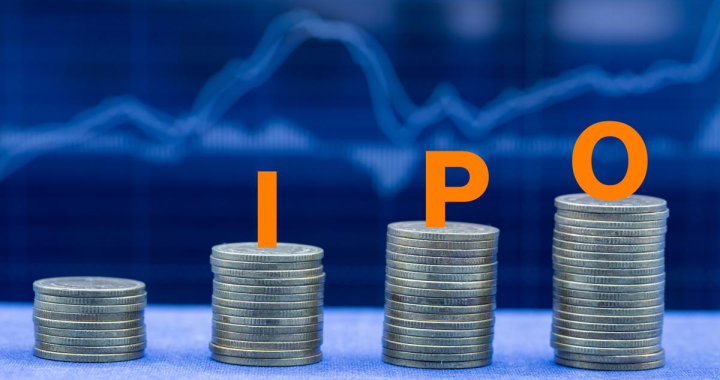September 27, 2023

Sites Wise Blog

# How to Calculate Your Fixed Deposit Interest Rate?Last Updated on July 21, 2021 by EricJones

Fixed deposits are an excellent way to meet your long-term financial goals. They are a universal favorite investment option. That is because you don’t have to worry about market risks.

You can earn interest on your FD while your money sits idle. Isn’t that an excellent way to grow your wealth? But how does the bank calculate your interest on FDs?

A fixed deposit (FD) is a savings deposit in which the interest rate for a specific period is predetermined. The deposit may be for only one time period or it may be payable at regular intervals after that, e.g., every year.

You can get overwhelmed at the thought of calculations. However, there’s nothing to worry about. We will make the process of calculating interest super simple for you.

### What is the Interest Rate for Fixed Deposit?

If you are wondering what the interest rate is for fixed deposits, it varies according to the financial institution. However, there is no significant variation—the current FD interest rates range from 5 percent to 6.7 percent per annum.

If you invest for a longer tenure, you will get higher rates of interest. Moreover, there are special interest rates for senior citizens. So if you are above 60 years of age, you can expect slightly higher rates than usual.

### Calculating Interest on Fixed Deposits

You can have two types of interest on your deposit- simple interest and compound interest. Let us take a look at each of them separately.

1. Simple Interest

It is calculated by the following formula-

PRT/100 is the principal amount. R is the rate of interest, and T is the tenure.

For instance, you invest a sum of Rs 10,000 for 2 years at 7% per annum. Your simple interest will be Rs 1400. And you will get a sum of Rs 11400 on maturity.

2. Compound Interest

Now, what about compound interest? Well, it might be a bit complicated. However, it will earn you higher interest.

Let us make it easy for you. Let us assume that you invest the same Rs 10000 for two years at the same interest rate. How will your interest get calculated?

For the first year, the bank will calculate simple interest. So at the end of one year, your interest will be Rs 700. And the amount will be Rs 10700.

This amount at the end of the first year will become the principal for the second year. So your interest for the second year will be Rs ( 1070017)/100 i.e., Rs 749. Thus you will get Rs 11449 at the end of two years.

Most banks and financial institutions calculate compound interest on your fixed deposits. However, they compound the interest quarterly rather than annually.

Final Word

Make sure you compare the rates offered by different institutions before investing your money. Doing your research will help you get a better interest rate. Moreover, you should check the credibility of the bank or NBFC while opening the fixed deposit account.

PNB Housing Finance offers fixed deposits at competitive rates. Furthermore, you can open your account online. So, why are you still waiting? Invest now and secure your future.

#### You may have missed5 min read

#### What is an IPO?4 min read

#### How Online Shopping Revolutionizes Kids Fashion in the UK4 min read

#### How to do Groceries Online Tips And Ideas4 min read